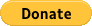## Exercise 1C Question 15

Is x^(2/3) = sqrt({x}^2) or is x^(2/3) = sqrt({x}^3) or is x^(2/3) > sqrt({x}^2) is or is x^(2/3) < sqrt({x}^2)

## Donate in USD